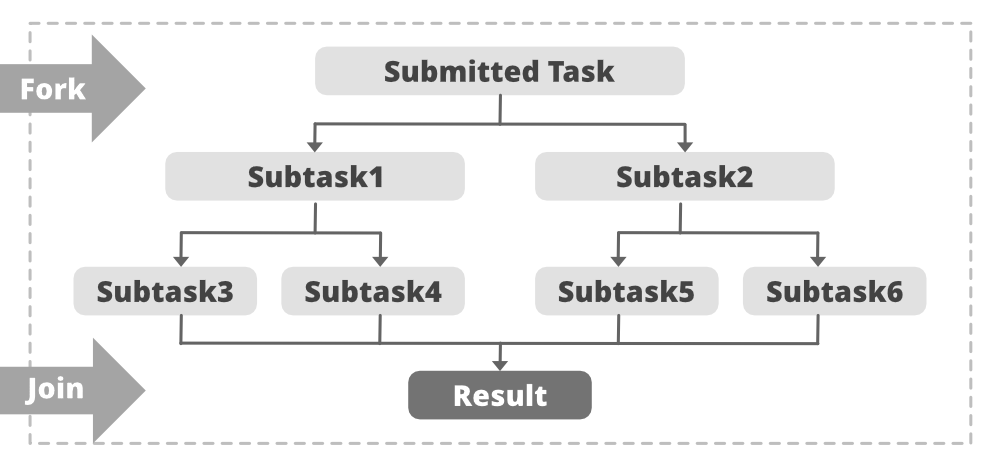Related Articles
ForkJoinPool Class in Java with Examples
• Last Updated : 03 Mar, 2021

The ForkJoinPool class is the center of the fork/join framework, which is an implementation of the ExectorService interface. ForkJoinPool class is an extension of the AbstractExecutorService class, and it implements the work-stealing algorithm (i.e., worker threads that run out of things to do can steal tasks from other threads that are still busy) of fork/join framework and can execute ForkJoinTask processes.

The ForkJoinPool class inherits the following methods from java.util.concurrent.AbstractExecutorService class:

• invokeAll()
• invokeAny()

The ForkJoinPool class inherits the following methods from Methods inherited from class java.lang.Object class:

• clone()
• equals()
• finalize()
• getClass()
• hashCode()
• notify()
• notifyAll()
• wait()

Syntax:

`public class ForkJoinPool extends AbstractExecutorService  `

Fork: Fork step splits the task into smaller subtasks and these tasks are executed concurrently.

Join: After the execution of the subtasks, the task may join all the results into one result.

This is illustrated in the diagram below:Example:

getActiveThreadCount(): This method returns an estimated number of threads that are currently stealing or executing tasks. It may overestimate the number of active threads.

Syntax

`public int getActiveThreadCount()`

## Java

 `// Java program to demonstrate the``// Implementation of getActiveThreadCount()`` ` `import` `java.util.ArrayList;``import` `java.util.List;``import` `java.util.concurrent.ForkJoinPool;``import` `java.util.concurrent.RecursiveAction;``class` `NewTask ``extends` `RecursiveAction ``{``    ``private` `long` `Load = ``0``;``     ` `    ``public` `NewTask(``long` `Load) { ``this``.Load = Load; }`` ` `    ``protected` `void` `compute()``    ``{``        ``// fork tasks into smaller subtasks``        ``List subtasks = ``new` `ArrayList();``        ``subtasks.addAll(createSubtasks());``         ` `        ``for` `(RecursiveAction subtask : subtasks) {``            ``subtask.fork();``        ``}``    ``}``     ` `    ``// function to create and add subtasks``    ``private` `List createSubtasks()``    ``{``        ``// create subtasks``        ``List subtasks = ``new` `ArrayList();``        ``NewTask subtask1 = ``new` `NewTask(``this``.Load / ``2``);``        ``NewTask subtask2 = ``new` `NewTask(``this``.Load / ``2``);``        ``NewTask subtask3 = ``new` `NewTask(``this``.Load / ``2``);``         ` `        ``// to add the subtasks``        ``subtasks.add(subtask1);``        ``subtasks.add(subtask2);``        ``subtasks.add(subtask3);``         ` `        ``return` `subtasks;``    ``}``}``public` `class` `JavaForkJoingetActivethreadcountExample1 {``    ``public` `static` `void` `main(``final` `String[] arguments)``        ``throws` `InterruptedException``    ``{``        ``// get no. of available core available``        ``int` `proc = Runtime.getRuntime().availableProcessors();``         ` `        ``System.out.println(``"Number of available core in the processor is: "``            ``+ proc);``             ` `        ``// get no. of threads active``        ``ForkJoinPool Pool = ForkJoinPool.commonPool();``         ` `        ``System.out.println(``"Number of active thread before invoking: "``            ``+ Pool.getActiveThreadCount());``             ` `        ``NewTask t = ``new` `NewTask(``400``);``         ` `        ``Pool.invoke(t);``         ` `        ``System.out.println(``"Number of active thread after invoking: "``            ``+ Pool.getActiveThreadCount());``        ``System.out.println(``"Common Pool Size is: "``                           ``+ Pool.getPoolSize());``    ``}``}`
Output
```Number of available core in the processor is: 4
Number of active thread before invoking: 0
Number of active thread after invoking: 3
Common Pool Size is: 3```

### Methods of ForkJoinPool Class

Attention reader! Don’t stop learning now. Get hold of all the important Java Foundation and Collections concepts with the Fundamentals of Java and Java Collections Course at a student-friendly price and become industry ready. To complete your preparation from learning a language to DS Algo and many more,  please refer Complete Interview Preparation Course.

My Personal Notes arrow_drop_up